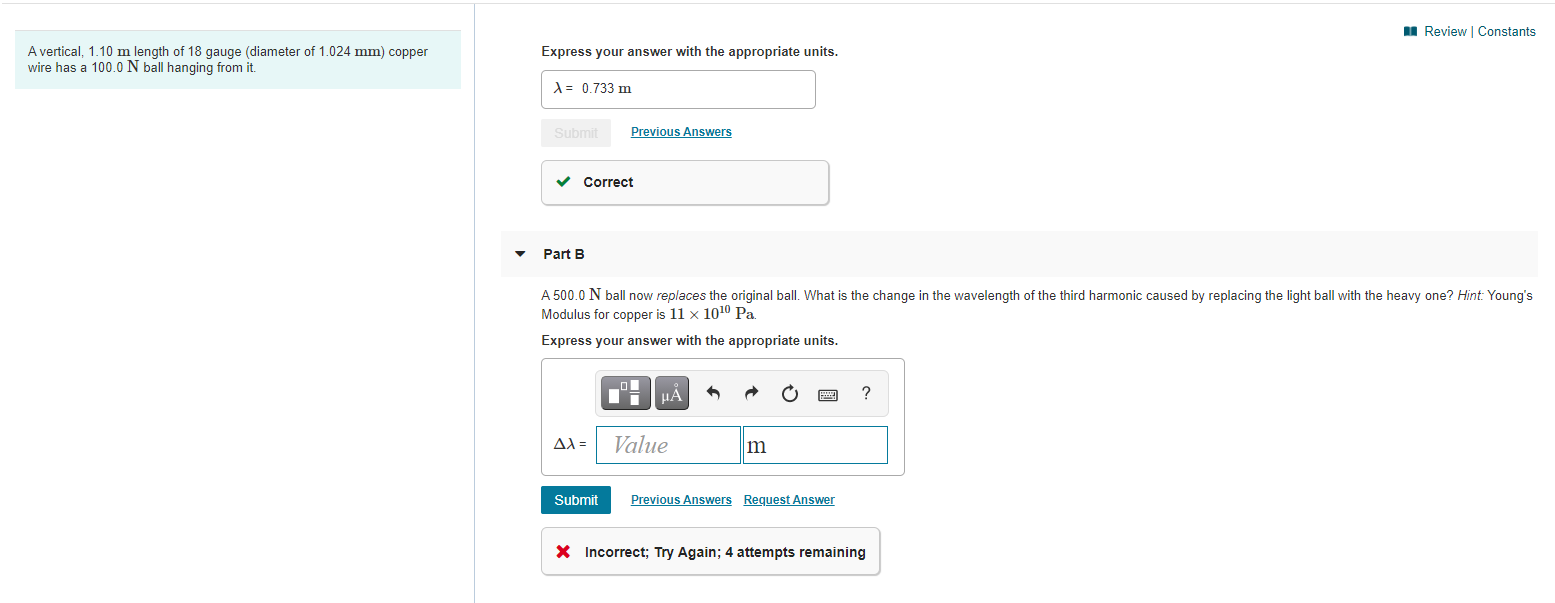# Question Solved1 AnswerA Review Constants A vertical, 1.10 m length of 18 gauge (diameter of 1.024 mm) copper wire has a 100.0 N ball hanging from it. Express your answer with the appropriate units. X = 0.733 m Submit Previous Answers ✓ Correct Part B A 500.0 N ball now replaces the original ball. What is the change in the wavelength of the third harmonic caused by replacing the light ball with the heavy one? Hint Young's Modulus for copper is 11 x 100 Pa Express your answer with the appropriate units. UA ? Δλ = Value m Submit Previous Answers Request Answer X Incorrect; Try Again; 4 attempts remainingTranscribed Image Text: A Review Constants A vertical, 1.10 m length of 18 gauge (diameter of 1.024 mm) copper wire has a 100.0 N ball hanging from it. Express your answer with the appropriate units. X = 0.733 m Submit Previous Answers ✓ Correct Part B A 500.0 N ball now replaces the original ball. What is the change in the wavelength of the third harmonic caused by replacing the light ball with the heavy one? Hint Young's Modulus for copper is 11 x 100 Pa Express your answer with the appropriate units. UA ? Δλ = Value m Submit Previous Answers Request Answer X Incorrect; Try Again; 4 attempts remaining
More
Transcribed Image Text: A Review Constants A vertical, 1.10 m length of 18 gauge (diameter of 1.024 mm) copper wire has a 100.0 N ball hanging from it. Express your answer with the appropriate units. X = 0.733 m Submit Previous Answers ✓ Correct Part B A 500.0 N ball now replaces the original ball. What is the change in the wavelength of the third harmonic caused by replacing the light ball with the heavy one? Hint Young's Modulus for copper is 11 x 100 Pa Express your answer with the appropriate units. UA ? Δλ = Value m Submit Previous Answers Request Answer X Incorrect; Try Again; 4 attempts remaining